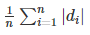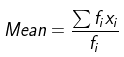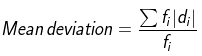• +91 9971497814
• info@interviewmaterial.com

# RD Chapter 32- Statistics Ex-32.2 Interview Questions Answers

### Related Subjects

Question 1 :

Calculate the meandeviation from the median of the following frequency distribution:

 Heights in inches 58 59 60 61 62 63 64 65 66 No. of students 15 20 32 35 35 22 20 10 8

To find the mean deviation from the median, firstly let uscalculate the median.

We know, Median is the Middle term,

So, Median = 61

Let xi =Heights in inches

And, fi = Number of students

 xi fi Cumulative Frequency |di| = |xi – M| = |xi – 61| fi |di| 58 15 15 3 45 59 20 35 2 40 60 32 67 1 32 61 35 102 0 0 62 35 137 1 35 63 22 159 2 44 64 20 179 3 60 65 10 189 4 40 66 8 197 5 40 N = 197 Total = 336

N=197

MD== 1/197 × 336

= 1.70

Themean deviation is 1.70.

Question 2 :

The number oftelephone calls received at an exchange in 245 successive on2-minute intervalsis shown in the following frequency distribution:

 Number of calls 0 1 2 3 4 5 6 7 Frequency 14 21 25 43 51 40 39 12

Compute the meandeviation about the median.

To find the mean deviation from the median, firstly let uscalculate the median.

We know, Median is the even term, (3+5)/2 = 4

So, Median = 8

Let xi =Number of calls

And, fi = Frequency

 xi fi Cumulative Frequency |di| = |xi – M| = |xi – 61| fi |di| 0 14 14 4 56 1 21 35 3 63 2 25 60 2 50 3 43 103 1 43 4 51 154 0 0 5 40 194 1 40 6 39 233 2 78 7 12 245 3 36 Total = 366 Total = 245

N = 245

MD== 1/245 × 336

= 1.49

Themean deviation is 1.49.

Question 3 :

Calculate the meandeviation about the median of the following frequency distribution:

 xi 5 7 9 11 13 15 17 fi 2 4 6 8 10 12 8

To find the mean deviation from the median, firstly let uscalculate the median.

We know, N = 50

Median = (50)/2 = 25

So, the median Corresponding to 25 is 13

 xi fi Cumulative Frequency |di| = |xi – M| = |xi – 61| fi |di| 5 2 2 8 16 7 4 6 6 24 9 6 12 4 24 11 8 20 2 16 13 10 30 0 0 15 12 42 2 24 17 8 50 4 32 Total = 50 Total = 136

N = 50

MD== 1/50 × 136

= 2.72

Themean deviation is 2.72.

Question 4 :

Find the meandeviation from the mean for the following data:

(i)

 xi 5 7 9 10 12 15 fi 8 6 2 2 2 6

(ii)

 xi 5 10 15 20 25 fi 7 4 6 3 5

(iii)

 xi 10 30 50 70 90 fi 4 24 28 16 8

(i)

To find the mean deviation from the mean, firstly let uscalculate the mean.

By using the formula,xi fi Cumulative Frequency (xifi) |di| = |xi – Mean| fi |di| 5 8 40 4 32 7 6 42 2 12 9 2 18 0 0 10 2 20 1 2 12 2 24 3 6 15 6 90 6 36 Total = 26 Total = 234 Total = 88= 234/26

= 9= 88/26

= 3.3

Themean deviation is 3.3

(ii)

To find the mean deviation from the mean, firstly let uscalculate the mean.

By using the formula,xi fi Cumulative Frequency (xifi) |di| = |xi – Mean| fi |di| 5 7 35 9 63 10 4 40 4 16 15 6 90 1 6 20 3 60 6 18 25 5 125 11 55 Total = 25 Total = 350 Total = 158= 350/25

= 14= 158/25

= 6.32

Themean deviation is 6.32

(iii)

To find the mean deviation from the mean, firstly let uscalculate the mean.

By using the formula,xi fi Cumulative Frequency (xifi) |di| = |xi – Mean| fi |di| 10 4 40 40 160 30 24 720 20 480 50 28 1400 0 0 70 16 1120 20 320 90 8 720 40 320 Total = 80 Total = 4000 Total = 1280= 4000/80

= 50= 1280/80

= 16

Themean deviation is 16

Question 5 :

Find the meandeviation from the median for the following data :

(i)

 xi 15 21 27 30 fi 3 5 6 7

(ii)

 xi 74 89 42 54 91 94 35 fi 20 12 2 4 5 3 4

(iii)

 Marks obtained 10 11 12 14 15 No. of students 2 3 8 3 4

(i)

To find the mean deviation from the median, firstly let uscalculate the median.

We know, N = 21

Median = (21)/2 = 10.5

So, the median Corresponding to 10.5 is 27

 xi fi Cumulative Frequency |di| = |xi – M| fi |di| 15 3 3 15 45 21 5 8 9 45 27 6 14 3 18 30 7 21 0 0 Total = 21 Total = 46 Total = 108

N = 21

MD== 1/21 × 108

= 5.14

Themean deviation is 5.14

(ii)

To find the mean deviation from the median, firstly let uscalculate the median.

We know, N = 50

Median = (50)/2 = 25

So, the median Corresponding to 25 is 74

 xi fi Cumulative Frequency |di| = |xi – M| fi |di| 74 20 4 39 156 89 12 6 32 64 42 2 10 20 80 54 4 30 0 0 91 5 42 15 180 94 3 47 17 85 35 4 50 20 60 Total = 50 Total = 189 Total = 625

N = 50

MD== 1/50 × 625

= 12.5

Themean deviation is 12.5

(iii)

To find the mean deviation from the median, firstly let uscalculate the median.

We know, N = 20

Median = (20)/2 = 10

So, the median Corresponding to 10 is 12

 xi fi Cumulative Frequency |di| = |xi – M| fi |di| 10 2 2 2 4 11 3 5 1 3 12 8 13 0 0 14 3 16 2 6 15 4 20 3 12 Total = 20 Total = 25

N = 20

MD== 1/20 × 25

= 1.25

Themean deviation is 1.25

Todays Deals### RD Chapter 32- Statistics Ex-32.2 Contributorskrishan

Name:
Email:

# Latest News# 9000 interview questions in different categories